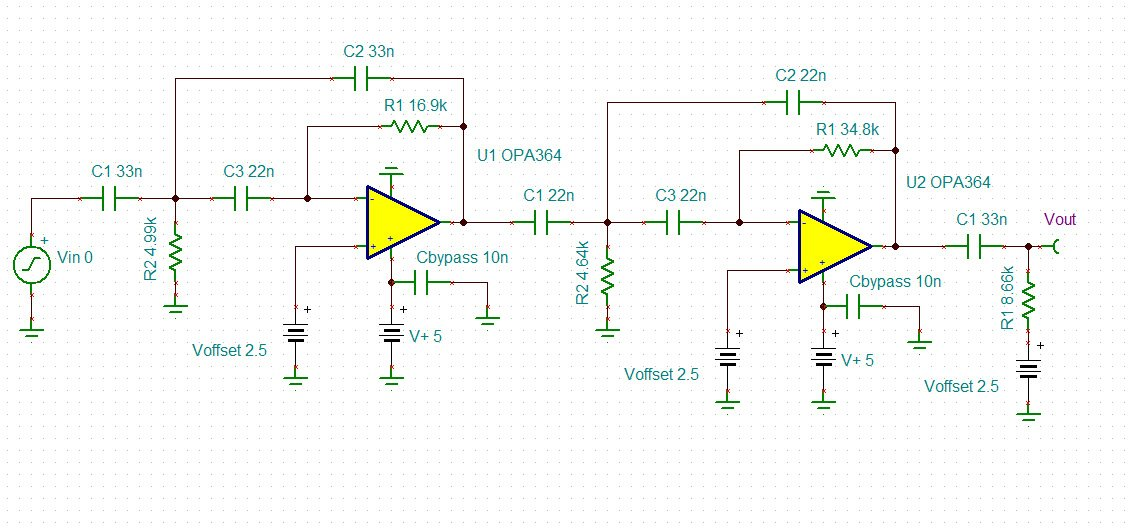# Find Poles and Zeros of Circuit by Inspection

Ing. Cristoforo Baldoni

In this article we ‘ll see how to recognize the number of poles and zeros of a transfer function simply by inspection, also of a large linear network, avoiding to calculate the analytical expression of the transfer function.  After reading this article, you ‘ll be able to determine the number of poles at first glance . Once set the output, you ‘ll also be able to determine the number of zeros by inspection and calculate the exact symbolic transfer function, the exact values of zeros and poles with simple software tool available for free. Using the SPICE analysis, we ‘ll verify the results found. The purpose of this article is to explore the concept of poles and zeros of a transfer function, their phisical meaning, and provide useful analysis tool for analog circuit designers and control systems engineers.

How many poles has the following network?